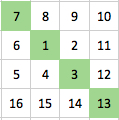#### You may also like### Prompt Cards

These two group activities use mathematical reasoning - one is numerical, one geometric.### Consecutive Numbers

An investigation involving adding and subtracting sets of consecutive numbers. Lots to find out, lots to explore.### Exploring Wild & Wonderful Number Patterns

EWWNP means Exploring Wild and Wonderful Number Patterns Created by Yourself! Investigate what happens if we create number patterns using some simple rules.

# Diagonal in a Spiral

##### Age 7 to 11Challenge Level

This is the start of a spiral, starting with 1 and then moving clockwise.
For this challenge we're interested in the upper-left to lower-right diagonal, shown in green. Here is the starting example:#### Challenge 1

Find the numbers that would be in this green upper-left to lower-right diagonal for a spiral going up to 144 instead of just 16.

#### Challenge 2

The small example above shows 7, 1, 3, 13 as the diagonal.
Now that you have a bigger diagonal going beyond 100 you need to deal with all the numbers in that diagonal in order from upper-left to lower-right.
You'll need to add the diagonal numbers in threes in order as they move through the square.
In the small example it would be 7 + 1 + 3 = 11 and 1 + 3 + 13 = 17. So your new numbers would be 11 and 17.
The totals you get for each three will give you a new set of numbers to use for Challenge 3.

#### Challenge 3a

You now need to use the numbers you got from adding the diagonal up in threes.
Use these numbers to make a total that has a 2 as the ones digit. You can only use a number once in any addition.
Do this in as many different ways as possible.

#### Challenge 3b

Do the same as in Challenge 3a but now the ones digit has to be an 8.
How many different ways are possible?